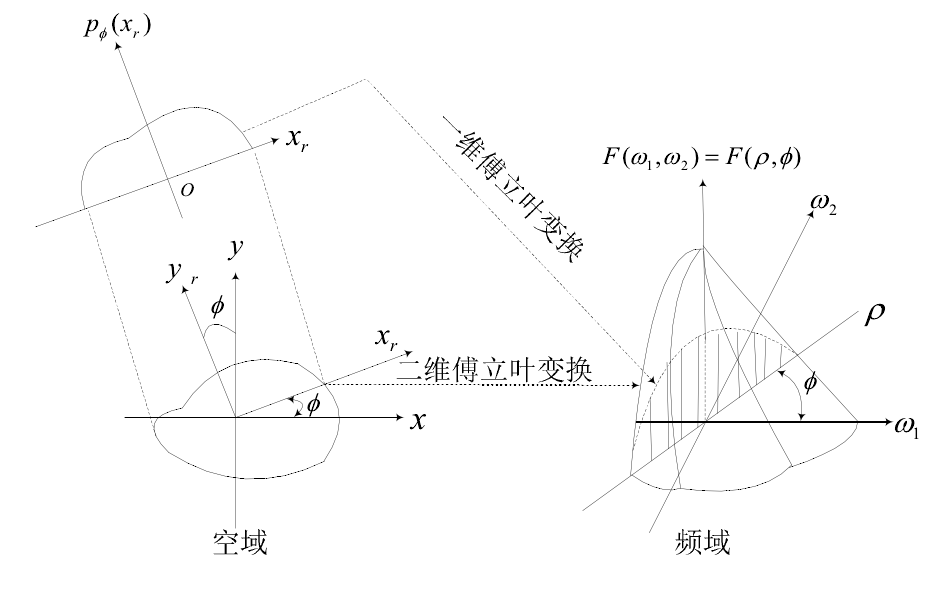• 滤波反投影重建算法（FBP）实现及应用（matlab）
2021-04-18 04:41:18

滤波反投影重建算法实现及应用(matlab)

1. 滤波反投影重建算法原理

滤波反投影重建算法常用在CT成像重建中，背后的数学原理是傅立叶变换：对投影的一维傅立叶变换等效于对原图像进行二维的傅立叶变换。(傅立叶中心切片定理)

CT重建算法大致分为解析重建算法和迭代重建算法，随着CT技术的发展，重建算法也变得多种多样，各有各的有特点。本文使用目前应用最广泛的重建算法——滤波反投影算法(FBP)作为模型的基础算法。FBP算法是在傅立叶变换理论基础之上的一种空域处理技术。它的特点是在反投影前将每一个采集投影角度下的投影进行卷积处理，从而改善点扩散函数引起的形状伪影，重建的图像质量较好。上图应可以清晰的描述傅立叶中心切片定理的过程：对投影的一维傅立叶变换等效于对原图像进行二维的傅立叶变换

傅立叶切片定理的意义在于，通过投影上执行傅立叶变换，可以从每个投影中得到二维傅立叶变换。从而投影图像重建的问题，可以按以下方法进行求解：采集不同时间下足够多的投影(一般为180次采集)，求解各个投影的一维傅立叶变换，将上述切片汇集成图像的二维傅立叶变换，再利用傅立叶反变换求得重建图像。

投影相关知识请参考fbp的matlab实现

2. 滤波反投影重建算法过程(以平行束为例)

投影重建的过程是，先把投影由线阵探测器上获得的投影数据进行一次一维傅立叶变换，再与滤波器函数进行卷积运算，得到各个方向卷积滤波后的投影数据；然后把它们沿各个方向进行反投影，即按其原路径平均分配到每一矩阵单元上，进行重叠后得到每一矩阵单元的CT值；再经过适当处理后得到被扫描物体的断层图像

算法步骤如下：

1. 将原始投影进行一次一维傅立叶变换

2. 设计合适的滤波器，在φ_i的角度下将得到原始投影p(x_r,φ_i)进行卷积滤波，得到滤波后的投影。

3. 将滤波后的投影进行反投影，得到满足x_r=r cos⁡((θ - φ_i))方向上的原图像的密度。

4. 将所有反投影进行叠加，得到重建后的投影。

3. 滤波器(滤波函数)和内插函数的选取

由于直接使用反投影算法会存在两个对实验结果影响很不好的因素：

不准确的数据重建图像就会产生各种伪影。

投影的数据是天然离散的，处理不当的话会产生很大的误差。

常见的滤波器有R-S滤波函数和S-L滤波函数。R-L滤波函数滤波计算简单，避免了大量的正弦、余弦计算，得到的采样序列分是分段现行的，并没有明显的降低图像质量，所以重建图像轮廓清楚，空间分辨率高。

常见的插值方法有最近邻插值和双线插值，最近邻插值即将离散点中间的缺失值用离它最近的整数处的投影值来替代。

4. FBP的matlab实现

使用R-L滤波器和最近邻插值方法

clc,clear;

%% 各个参数信息

%重建后图片像素个数

M=512;%建议先和接收传感器的个数一样

%旋转的角度 180次旋转

%投影为512行，180列的数据，列的数据对应每一次旋转，512个接收传感器

%由投影进行反变换

size(R,1);%512

% 设置快速傅里叶变换的宽度

width = 2^nextpow2(size(R,1));

%% 对投影做快速傅里叶变换并滤波

%傅立叶变换

proj_fft = fft(R, width);

% filter 滤波

% R-L是一种基础的滤波算法

filter = 2*[0:(width/2-1), width/2:-1:1]'/width;

% 滤波后结果 proj_filtered

proj_filtered = zeros(width,180);

for i = 1:180

proj_filtered(:,i) = proj_fft(:,i).*filter;

end

figure

subplot(1,2,1),imshow(proj_fft),title('傅立叶变换')

subplot(1,2,2),imshow(proj_filtered),title('傅立叶变换+滤波')

%% 逆快速傅里叶变换并反投影

% 逆快速傅里叶变换 proj_ifft

proj_ifft = real(ifft(proj_filtered));

figure,imshow(proj_ifft),title('逆傅立叶变换')

%反投影到x轴，y轴

fbp = zeros(M); % 假设初值为0

for i = 1:180

for x = 1:M

for y = 1:M

%{

%最近邻插值法

if t-size(R,1)/2

fbp(x,y)=fbp(x,y)+proj_ifft(round(t+size(R,1)/2),i);

end

%}

%最近邻插值法

t = round(t_temp) ;

if t>0 && t<=512

fbp(x,y)=fbp(x,y)+proj_ifft(t,i);

end

end

end

end

fbp = (fbp*pi)/180;%512x512 原图像每个像素位置的密度

xlswrite('problem2_origin.xlsx',fbp,'Sheet1');%将得到的重建后的图像数据写入

% 显示结果

figure,imshow(fbp),title('反投影变换后的图像')

其中每一个文件都有作用的说明，如需源文件请留言哈。(如有错误请指正)

fbp算法实现案例

github代码下载 别忘了给star哟~

参考文献：

【1】 范慧赟.CT 图像滤波反投影重建算法的研究[D].硕士学位论文,西北工业大学,2007.

【2】 余晓锷,龚剑,马建华等.CT 原理与技术[M].北京:科学出版社,2013,95-97.

【3】 毛小渊. 二维CT图像重建算法研究[D].南昌航空大学,2016.

【4】 洪虹. CT中金属伪影的校正研究[D].南方医科大学,2013.

【5】 范慧赟. CT图像滤波反投影重建算法的研究[D].西北工业大学,2007.

更多相关内容
• 其余为自己编写，通过两种不同插值的方法，实现直接反投影和滤波反投影，最终script实现3种不同投影个数的重建效果。 适合CT重建算法初学者调试学习，直观了解不同算法，不同投影个数引起的不同结果。 phantom 为一...
• 二维滤波反投影重建算法，旋转角度为0-180度，测试后发现略有角度偏移，希望各位指正。
• 对扁平构件的仿真结果表明:在射线源为锥束入射扫描方式下,通过分布在锥面上的ρ滤波器进行滤波反投影重建,得到的重建切片图像能够明显区分物体的边界及内部缺陷的边界和密度,空间分辨率可达到2 lp/ mm,密度分辨率...
• CT平行束滤波反投影重建程序，利用matlab自带pantom进行正向投影，然后通过滤波反投影恢复出图像
• 实验一平行束投影数据采集与滤波反投影重建实验实验报告，附有matlab源码
• 基于matlab的平行束滤波反投影重建算法。基于matlab的平行束滤波反投影重建算法
• 基于等距扇形束滤波反投影(FBP)算法推导了一种新的算法求导希尔伯特反投影(DHB)算法，研究了DHB算法在频域对投影的滤波特性。通过理论分析和实验验证，指出由于DHB滤波函数在高频段对于锐截止特性的改善，很大程度上...
• 为什么光有反投影不足以得到质量较好的图像，下面这个图像能很好的说明问题，图像的边缘模糊 二…中心切片定理 二维图像的中心切片定理指出：二维函数 f(x, y) 的投影 p(s) 之傅里叶变换 P(ω) 等于函数 f(x, y) 的...

一.为什么光有反投影不足以得到质量较好的图像，下面这个图像能很好的说明问题，图像的边缘模糊二…中心切片定理
二维图像的中心切片定理指出：二维函数 f(x, y) 的投影 p(s) 之傅里叶变换 P(ω) 等于函数 f(x, y) 的傅里叶变换 F(ωx, ωy) 沿与探测器平行的方向过原点的片段

1.一维连续傅里叶变换对：二维连续傅里叶变换对：2.根据中心切片的定义，如果探测器绕物体旋转至少180°，物体的二维傅里叶变换 ) , F （ωx, ωy) 所对应于探测器方向的中心片段就能覆盖整个傅里叶空间,也就是说，知道物体的二维的傅里叶变换，二维傅里叶反变换就可以恢复出物体3.中心切片定理的证明：三.FBP (Filtered Backprojection 先滤波后反投影) 算法
从上面的频域图来看，每一个角度的投影的傅里叶变换，是穿过频域坐标中心的一条直线，最后形成一个点散射的形状，中心片段在 ωx-ωy 平面的原点的密度高于在远离原点的区域的密度，而在傅里叶空间原点附近的区域是低频区域。对低频分量的过分加权导致图像变得模糊。为了消除模糊的效果，我们对傅里叶空间要进行加权矫正，使其密度均匀。因此使用了一个低频滤波器，|w|，抑制低频成分，提高图像的清晰度

近期补上剩下的部分

展开全文• 实验一平行束投影数据采集与滤波反投影重建实验宣贯.pdf
• 实验一平行束投影数据采集与滤波反投影重建实验[参照].pdf
• ct Matlab代码CT重建 Matlab代码，通过应用反投影，滤波反投影和卷积反投影来重建CT图像。
• 利用MATLAB实现滤波反投影技术，编写的一个程序。。。。
• 利用MATLAB实现了基于扇形滤波反投影重建算法，对学习反投影重建算法有一定的帮助，以及扇形滤波等知识
• 图像重建的基本思想，就是通过探测物体的投影数据，重建物体的实际内部...滤波反投影重建算法在反投影前将每一个采集投影角度下的投影进行卷积处理，从而改善点扩散函数引起的形状伪影，有效地改善了重建的图像质量。

欢迎关注 『youcans 的 OpenCV 例程 200 篇』 系列，持续更新中
欢迎关注 『youcans 的 OpenCV学习课』 系列，持续更新中

# 【youcans 的 OpenCV 例程 200 篇】112. 滤波反投影重建图像

## 7. 投影重建图像

图像重建的基本思想，就是通过探测物体的投影数据，重建物体的实际内部构造。

### 7.3 滤波反投影重建图像 （Filtered back projection）

直接由正弦图得到反投影图像，会存在严重的模糊，这是早期 CT 系统所存在的问题。

傅立叶中心切片定理表明，投影的一维傅立叶变换是得到投影区域的二维傅立叶变换的切片。滤波反投影重建算法在反投影前将每一个采集投影角度下的投影进行卷积处理，从而改善点扩散函数引起的形状伪影，有效地改善了重建的图像质量。

f ( x , y ) f(x,y) 表示需要重建的图像， F ( u , v ) F(u,v) 的二维傅里叶反变换是：
f ( x , y ) = ∫ − ∞ ∞ ∫ − ∞ ∞ F ( u , v ) e j 2 π ( u x + v y ) d u d v f(x,y) = \int^{\infty}_{-\infty} \int^{\infty}_{-\infty} F(u,v) e^{j 2 \pi (ux+vy)} dudv
利用傅里叶切片定理，可以得到：
f ( x , y ) = ∫ 0 π [ ∫ − ∞ ∞ ∣ ω ∣ G ( ω , θ ) e j 2 π ω ρ d ω ] d θ f(x,y) = \int^{\pi}_{0} \big[ \int^{\infty}_{-\infty} |\omega|G(\omega,\theta) e^{j 2 \pi \omega \rho} d\omega \big] d\theta
括号 [] 内部是一个一维傅里叶反变换，可以认为这是一个一维滤波器的传递函数。由于 ∣ ω ∣ |\omega| 是一个不可积的斜坡函数（Slope function），可以通过对斜坡加窗进行限制，典型地如汉明窗（Hamming window）、韩窗（Hann window）。

该式也可以使用空间卷积来实现：
f ( x , y ) = ∫ 0 π [ ∫ − ∞ ∞ ∣ ω ∣ G ( ω , θ ) e j 2 π ω ρ d ω ] d θ = ∫ 0 π [ ∫ − ∞ ∞ g ( ρ , θ ) s ( x c o s θ + y s i n θ − ρ ) d ρ ] d θ f(x,y) = \int^{\pi}_{0} \big[ \int^{\infty}_{-\infty} |\omega|G(\omega,\theta) e^{j 2 \pi \omega \rho} d\omega \big] d\theta \\ = \int^{\pi}_{0} \big[ \int^{\infty}_{-\infty} g(\rho,\theta) s(xcos \theta + ysin \theta - \rho) d\rho \big] d\theta \\
这表明，将对应的投影 g ( ρ , θ ) g(\rho, \theta) 与斜坡滤波器传递函数 s ( ρ ) s(\rho) 的傅里叶反变换进行卷积，可以得到角度 θ \theta 的各个反投影，整个反投影图像可以通过对所有反投影图像积分得到。

### 例程 9.25：滤波反投影重建图像

SL 滤波器是使用 Sinc 函数对斜坡滤波器进行截断产生，其离散形式为：
h S L ( n δ ) = − 2 π 2 δ 2 ( 4 ∗ n 2 − 1 ) ,   n = 0 , ± 1 , ± 2 , . . . h_{SL}(n \delta) = - \frac{2}{\pi ^2 \delta ^2 (4*n^2 -1)}, \ n=0,\pm 1,\pm 2,...
利用投影值和 SL 滤波器进行卷积，然后再进行反投影，就可以实现图像重建。

   # 9.25: 滤波反投影重建图像
from scipy import ndimage
channels = image.shape
for s in range(steps):
rotation = ndimage.rotate(image, -s * 180/steps, reshape=False).astype(np.float32)

channels = image.shape
backproject = np.zeros((steps, channels, channels))
for s in range(steps):
expandDims = np.expand_dims(image[:, s], axis=0)
repeat = expandDims.repeat(channels, axis=0)
backproject[s] = ndimage.rotate(repeat, s * 180/steps, reshape=False).astype(np.float32)

def SLFilter(N, d):  # SL 滤波器, Sinc 函数对斜坡滤波器进行截断
filterSL = np.zeros((N,))
for i in range(N):
filterSL[i] = - 2 / (np.pi**2 * d**2 * (4 * (i-N/2)**2 - 1))
return filterSL

channels = len(image)
backproject = np.zeros((steps, channels, channels))  # 反投影
filter = SLFilter(channels, 1)  # SL 滤波器
for s in range(steps):
value = image[:, s]  # 投影值
valueFiltered = np.convolve(filter, value, "same")  # 投影值和 SL 滤波器进行卷积
filterExpandDims = np.expand_dims(valueFiltered, axis=0)
filterRepeat = filterExpandDims.repeat(channels, axis=0)
backproject[s] = ndimage.rotate(filterRepeat, s * 180/steps, reshape=False).astype(np.float32)

# 读取原始图像
img1 = cv2.imread("../images/Fig0534a.tif", 0)  # flags=0 读取为灰度图像

# 雷登变换

# 雷登变换反投影重建图像

# 滤波反投影重建图像

plt.figure(figsize=(9, 7))
plt.subplot(231), plt.axis('off'), plt.title("origin image"), plt.imshow(img1, 'gray')  # 绘制原始图像
plt.subplot(234), plt.axis('off'), plt.title("origin image"), plt.imshow(img2, 'gray')
plt.tight_layout()
plt.show()（本节完）

版权声明：

youcans@xupt 原创作品，转载必须标注原文链接：(https://blog.csdn.net/youcans/article/details/123088438)

Crated：2022-2-22

欢迎关注 『youcans 的 OpenCV 例程 200 篇』 系列，持续更新中
欢迎关注 『youcans 的 OpenCV学习课』 系列，持续更新中

展开全文opencv python 图像处理 计算机视觉 算法
• 例子 ImageRecon 统一磁盘和统一同心磁盘的过滤反投影。 汉字。 互动事项 康普顿 放射性同位素生产 Mo-99的生产示例。 洗脱发生器的示例。...